## 最大流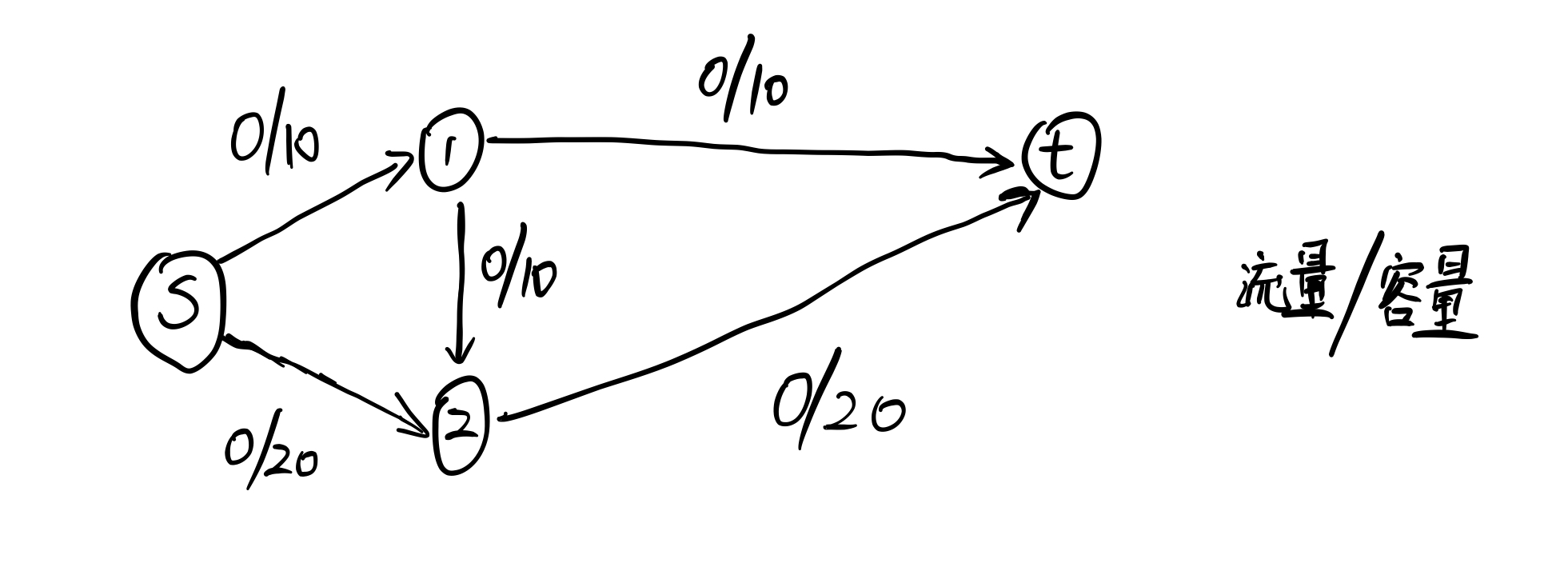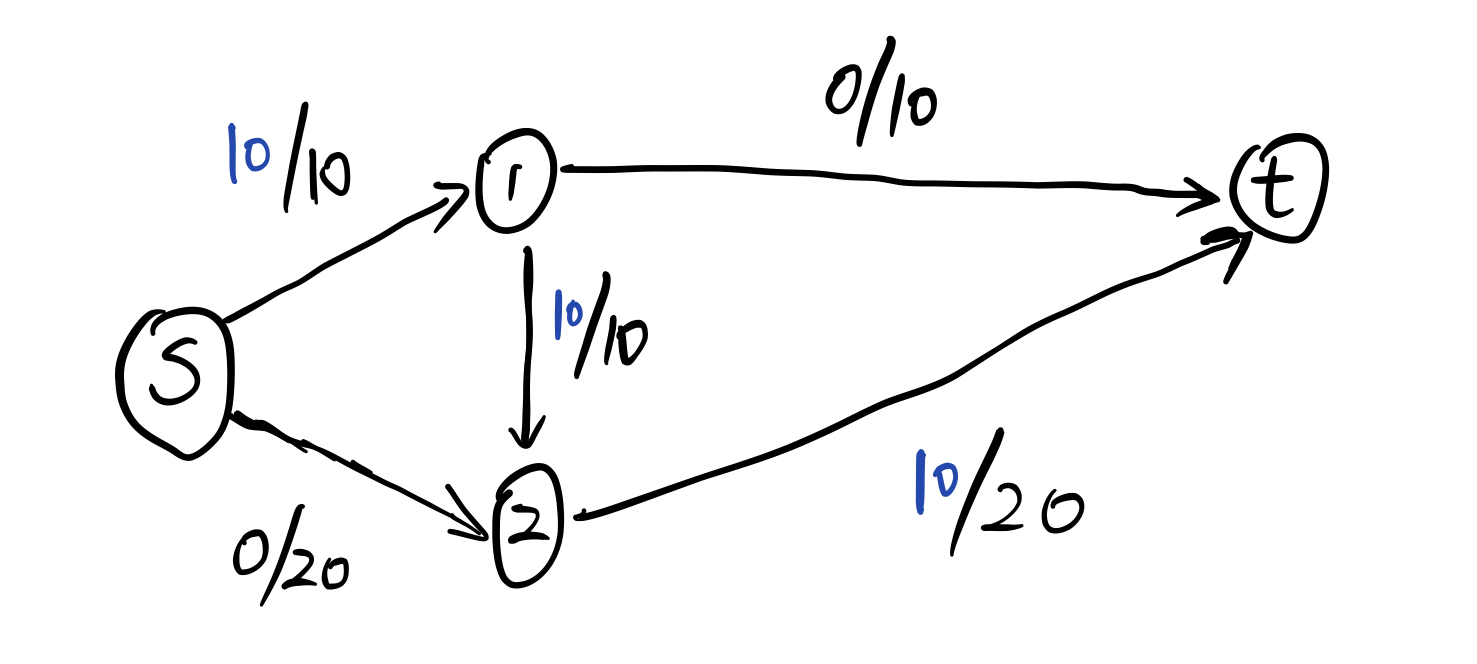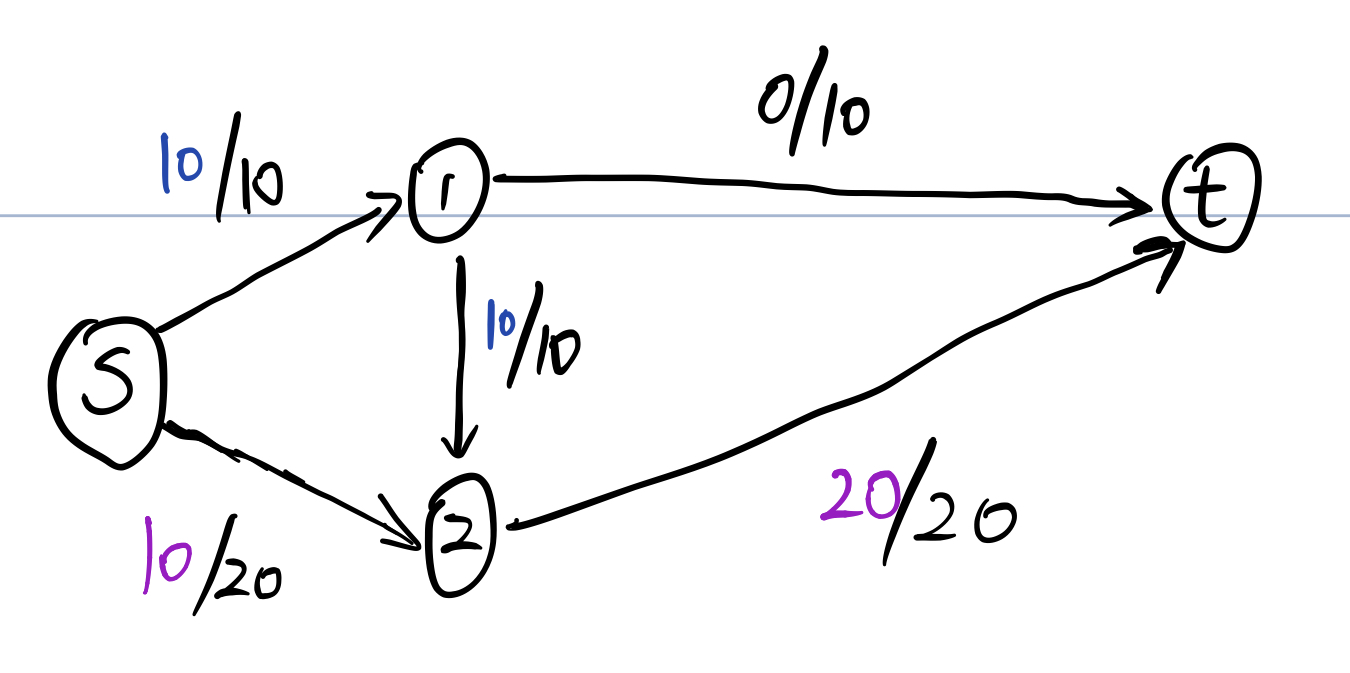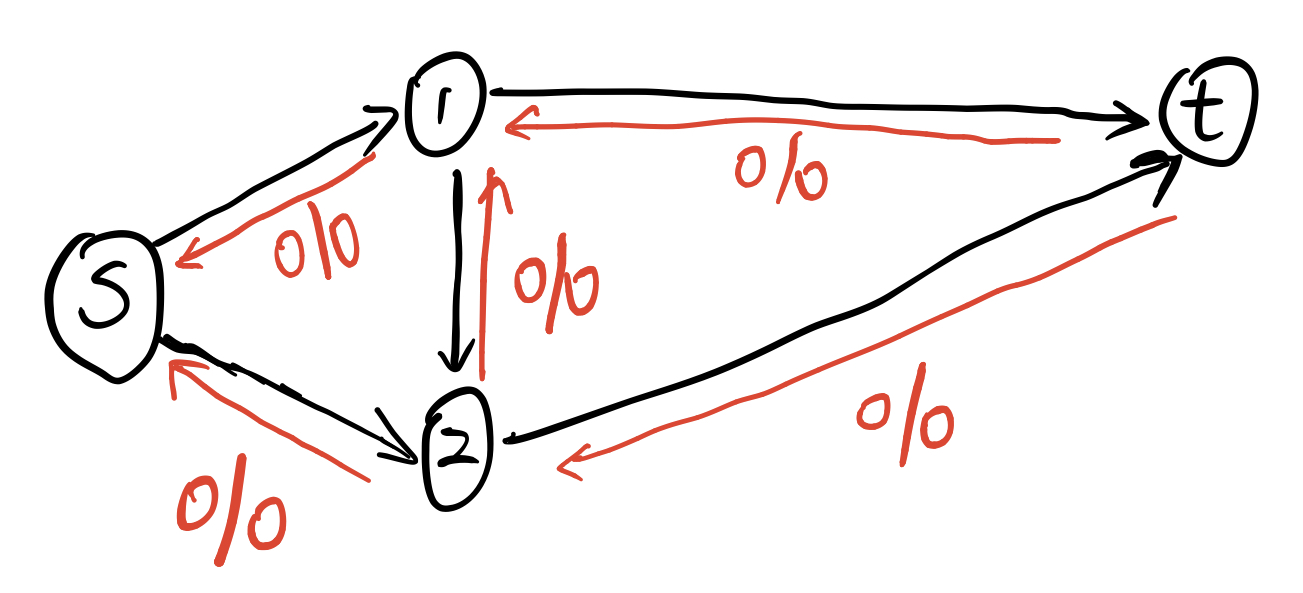### Edmond—Karp

struct Edge{
int from,to,cap,flow;
Edge(int u,int v,int c,int f): from(u),to(v),cap(c),flow(f) {}
};
struct EdmonsKarp{          //时间复杂度O(v*E*E)
int n,m;
vector<Edge> edges;     //边数的两倍
vector<int> G[maxn];    //邻接表，G[i][j]表示节点i的第j条边在e数组中的序号
int a[maxn];            //起点到i的可改进量
int p[maxn];            //最短路树上p的入弧编号

void init(int n){
for(int i=0;i<=n;i++) G[i].clear();
edges.clear();
}

edges.push_back(Edge(from,to,cap,0));
edges.push_back(Edge(to,from,0,0));     //反向弧
m = edges.size();
G[from].push_back(m-2);
G[to].push_back(m-1);
}

int Maxflow(int s,int t){
int flow = 0;
for(;;){
for (int i = 0; i <= n; i++) a[i] = 0;
queue<int> Q;
Q.push(s);
a[s] = INF;
while(!Q.empty()){
int x = Q.front();Q.pop();
for(int i=0;i<G[x].size();i++){
Edge& e = edges[G[x][i]];
if(!a[e.to] && e.cap > e.flow){     //!a[e.to] 是了保证不会回退和出现分叉
p[e.to] = G[x][i];      //记录边的编号
a[e.to] = min(a[x],e.cap - e.flow);
Q.push(e.to);
}
}
if(a[t]) break;         //走到汇点
}
if(!a[t]) break;            //没有一条增广路存在
for(int u=t;u != s;u = edges[p[u]].from){
edges[p[u]].flow += a[t];
edges[p[u]^1].flow -= a[t];
}
flow += a[t];
}
return flow;
}
};

### Dinic

const int maxn = 1e4 + 6;
const int INF = 0x3f3f3f3f;
struct Edge {
int u, v;
int cap, flow;
Edge(int u_, int v_, int cap_, int flow_)
: u(u_), v(v_), cap(cap_), flow(flow_) {}
};

struct Dinic {
int n, m, s, t;  //结点数，边数（包括反向弧），源点编号和汇点编号
vector<Edge> edges;  //边表。edge[e]和edge[e^1]互为反向弧
vector<int> G[maxn];  //邻接表，G[i][j]表示节点i的第j条边在e数组中的序号
bool vis[maxn];  // BFS使用
int d[maxn];     //从起点到i的距离
int cur[maxn];   //当前弧下标
void init(int n) {
this->n = n;
for (int i = 0; i <= n; i++) G[i].clear();
edges.clear();
}
int AddEdge(int from, int to, int cap) {
edges.emplace_back(from, to, cap, 0);
edges.emplace_back(to, from, 0, 0);
m = edges.size();
G[from].push_back(m - 2);
G[to].push_back(m - 1);
return m - 2;
}
bool BFS() {
for (int i = 0; i <= n; ++i) vis[i] = false, d[i] = 0;
queue<int> q;
q.push(s);
d[s] = 0;
vis[s] = 1;
while (!q.empty()) {
int x = q.front();
q.pop();
for (int i = 0, v; i < G[x].size(); i++) {
Edge& e = edges[G[x][i]];
v = e.v;
if (!vis[v] && e.cap > e.flow) {
vis[v] = 1;
d[v] = d[x] + 1;
q.push(v);
}
}
}
return vis[t];
}
int DFS(int x, int a) {
if (x == t || a == 0) return a;
int flow = 0, f;
for (int &i = cur[x], v; i < G[x].size(); i++) {  //从上次考虑的弧
Edge& e = edges[G[x][i]];
v = e.v;
if (d[x] + 1 == d[v] && (f = DFS(v, min(a, e.cap - e.flow))) > 0) {
e.flow += f;
edges[G[x][i] ^ 1].flow -= f;
flow += f;
a -= f;
if (a == 0) break;
}
}
return flow;
}
int Maxflow(int s, int t) {
this->s = s, this->t = t;
int flow = 0;
while (BFS()) {
for (int i = 0; i <= n; i++) cur[i] = 0;
flow += DFS(s, INF);
}
return flow;
}

int edge_id[maxn];  // 每条边的编号
vector<int> cut;    // 割边的编号
void findCut(int m) {
cut.clear();
for (int i = 0; i <= n; i++) vis[i] = 0;
queue<int> q;
q.push(s);
vis[s] = 1;
while (!q.empty()) {
int u = q.front();
q.pop();
for (auto &id : G[u]) {
auto &e = edges[id];
if (!vis[e.v] && e.cap > e.flow) {
vis[e.v] = 1;
q.push(e.v);
}
}
}
for (int i = 0; i < m; i++) {
auto &e = edges[edge_id[i]];
if (vis[e.u] == vis[e.v]) continue;
if (e.cap == e.flow) cut.push_back(i + 1);  // 1-index
}
}
};

### ISAP

const int maxn = "Edit";
struct ISAP {
int n, m, s, t;  //结点数，边数（包括反向弧），源点编号和汇点编号
vector<Edge> edges;  //边表。edges[e]和edges[e^1]互为反向弧
vector<int> G[maxn];  //邻接表，G[i][j]表示结点i的第j条边在e数组中的序号
bool vis[maxn];  // BFS使用
int d[maxn];     //起点到i的距离
int cur[maxn];   //当前弧下标
int p[maxn];     //可增广路上的一条弧
int num[maxn];   //距离标号计数
void init(int n) {
this->n = n;
for (int i = 0; i < n; i++) G[i].clear();
edges.clear();
}
void AddEdge(int from, int to, int cap) {
edges.emplace_back(from, to, cap, 0);
edges.emplace_back(to, from, 0, 0);
int m = edges.size();
G[from].push_back(m - 2);
G[to].push_back(m - 1);
}
int Augumemt() {
int x = t, a = INF;
while (x != s) {
Edge& e = edges[p[x]];
a = min(a, e.cap - e.flow);
x = edges[p[x]].from;
}
x = t;
while (x != s) {
edges[p[x]].flow += a;
edges[p[x] ^ 1].flow -= a;
x = edges[p[x]].from;
}
return a;
}
void BFS() {
for (int i = 0; i <= n; i++) vis[i] = 0;
for (int i = 0; i <= n; i++) d[i] = 0;
queue<int> q;
q.push(t);
d[t] = 0;
vis[t] = 1;
while (!q.empty()) {
int x = q.front();
q.pop();
int len = G[x].size();
for (int i = 0; i < len; i++) {
Edge& e = edges[G[x][i] ^ 1];
if (!vis[e.from] && e.cap > e.flow) {
vis[e.from] = 1;
d[e.from] = d[x] + 1;
q.push(e.from);
}
}
}
}
int Maxflow(int s, int t) {
this->s = s;
this->t = t;
int flow = 0;
BFS();
for (int i = 0; i <= n; i++) num[i] = 0;
for (int i = 0; i < n; i++)
if (d[i] < INF) num[d[i]]++;
int x = s;
for (int i = 0; i <= n; i++) cur[i] = 0;
while (d[s] < n) {
if (x == t) {
flow += Augumemt();
x = s;
}
int ok = 0;
for (int i = cur[x]; i < G[x].size(); i++) {
Edge& e = edges[G[x][i]];
if (e.cap > e.flow && d[x] == d[e.to] + 1) {
ok = 1;
p[e.to] = G[x][i];
cur[x] = i;
x = e.to;
break;
}
}
if (!ok)  // Retreat
{
int m = n - 1;
for (int i = 0; i < G[x].size(); i++) {
Edge& e = edges[G[x][i]];
if (e.cap > e.flow) m = min(m, d[e.to]);
}
if (--num[d[x]] == 0) break;  // gap优化
num[d[x] = m + 1]++;
cur[x] = 0;
if (x != s) x = edges[p[x]].from;
}
}
return flow;
}
};

## 最小费用最大流

### 模板

struct Edge{
int from,to,cap,flow,cost;
Edge(int u,int v,int c,int f,int w):from(u),to(v),cap(c),flow(f),cost(w) {}
};

struct MCMF{
int n,m;
vector<Edge> edges;
vector<int> G[maxn];
int inq[maxn];      //是否在队列中
int d[maxn];        //bellmanford 到源点距离
int p[maxn];        //上一条弧
int a[maxn];        //可改进量

void init(int n){
this-> n = n;
for(int i=0;i<=n;i++) G[i].clear();
edges.clear();
}

void AddEdge(int from,int to,int cap,int cost){
edges.push_back(Edge(from,to,cap,0,cost));
edges.push_back(Edge(to,from,0,0,-cost));
m = edges.size();
G[from].push_back(m-2);
G[to].push_back(m-1);
}

bool BellmanFord(int s,int t,int& flow,ll& cost){
for(int i=0;i<=n;i++) d[i] = INF;
for (int i = 0; i <= n; i++) inq[i] = 0;
d[s] = 0; inq[s] = 1; p[s] = 0; a[s] = INF;
queue<int> Q;
Q.push(s);
while(!Q.empty()){
int u = Q.front(); Q.pop();
inq[u] = 0;
for(int i=0;i<G[u].size();i++){
Edge& e = edges[G[u][i]];
if(e.cap > e.flow && d[e.to] > d[u] + e.cost){
d[e.to] = d[u] + e.cost;
p[e.to] = G[u][i];
a[e.to] = min(a[u], e.cap - e.flow);
if(!inq[e.to]) {Q.push(e.to); inq[e.to] = 1;}
}
}
}
if(d[t] == INF) return false;    // 当没有可增广的路时退出
flow += a[t];
cost += (ll)d[t] * (ll)a[t];
for(int u=t; u!= s; u = edges[p[u]].from){
edges[p[u]].flow += a[t];
edges[p[u]^1].flow -= a[t];
}
return true;
}

int MincostMaxflow(int s,int t,ll& cost){
int flow = 0; cost = 0;
while(BellmanFord(s,t,flow,cost));
return flow;
}
};

## 经典建图

### 无源汇可行流（循环流）

• 对于 $a[i] > 0$，建立 $(ss, i)$ 容量为 $a[i]$ 的边。

$a[i] > 0$ 表示 $i$ 点流入的流量多于流出的流量，那我们需要在残余网络上表示出来这个多余的流量，因此建立 $(ss,i)$ 的边，表示 $i$ 点会多进入一些流量。如果最后这个边可以跑满，说明多余的流量可以找到出路，从而可以达到流量平衡。

• 对于 $a[i] < 0$， 建立 $(i,tt)$ 容量为 $-a[i]$ 的边

• 对于原图中的边 $(u, v, low, high)$，建立 $(u,v)$ 容量为 $high - low$ 的边。

• 跑 $ss$ 到 $tt$ 的最大流，如果从 $ss$ 出去的边（进入 $tt$ 的边）全部满流，则证明有解，否则无解。

### 有源汇可行流

• 设源点为 $s$，汇点为 $t$。
• 建立一条 $(t,s)$ 容量为无穷大的边。
• 再按照无源汇可行流的方式建图。
• 跑 $ss$ 到 $tt$ 的最大流，如果从 $ss$ 出去的边（进入 $tt$ 的边）全部满流，则证明有解，否则无解。

### 有源汇上下界最大流

• 按照有源汇可行流的方式建图。
• 跑 $ss$ 到 $tt$ 的最大流，如果从 $ss$ 出去的边（进入 $tt$ 的边）全部满流，则证明有解，否则无解。
• 再跑 $s$ 到 $t$ 的最大流，得到的流量即为答案。

• 会不会有的边退流之后就不满足最后的流量平衡了？

• 是否需要去掉源点汇点和 $(t,s)$ 这条边？

### 有源汇上下界最小流

• 按照无源汇可行流进行建图
• 跑 $ss$ 到 $tt$ 的最大流
• 建立 $(t,s)$
• 再跑 $ss$ 到 $tt$ 的最大流，$(t,s)$ 这条边上的流量就是答案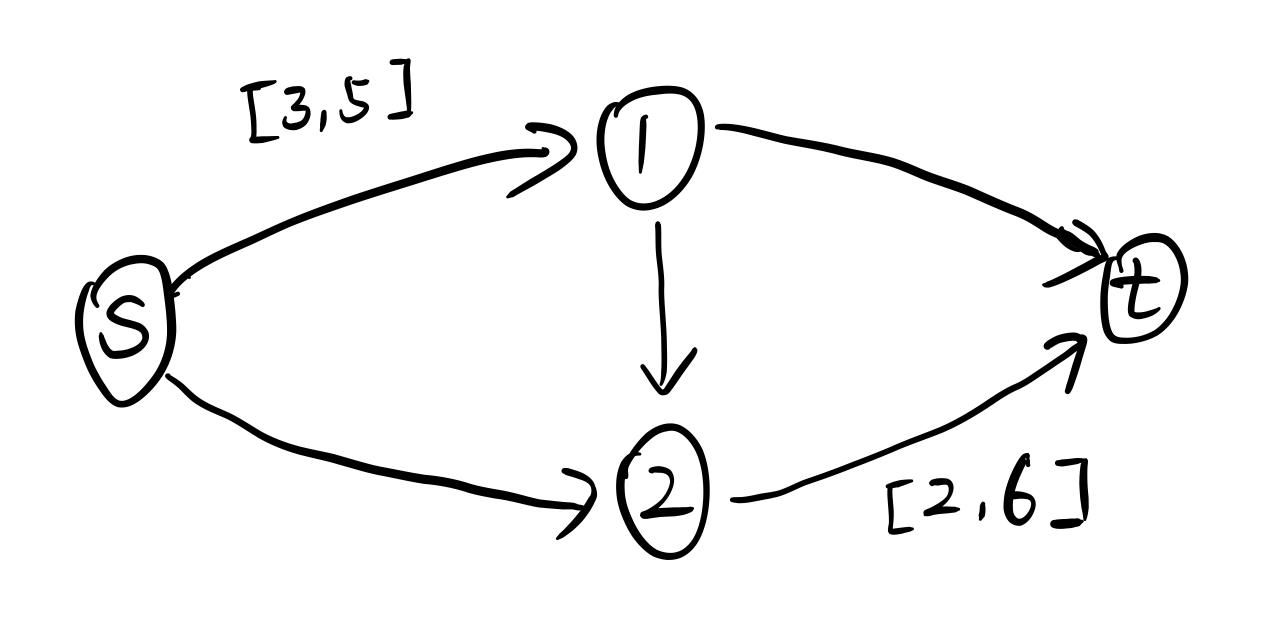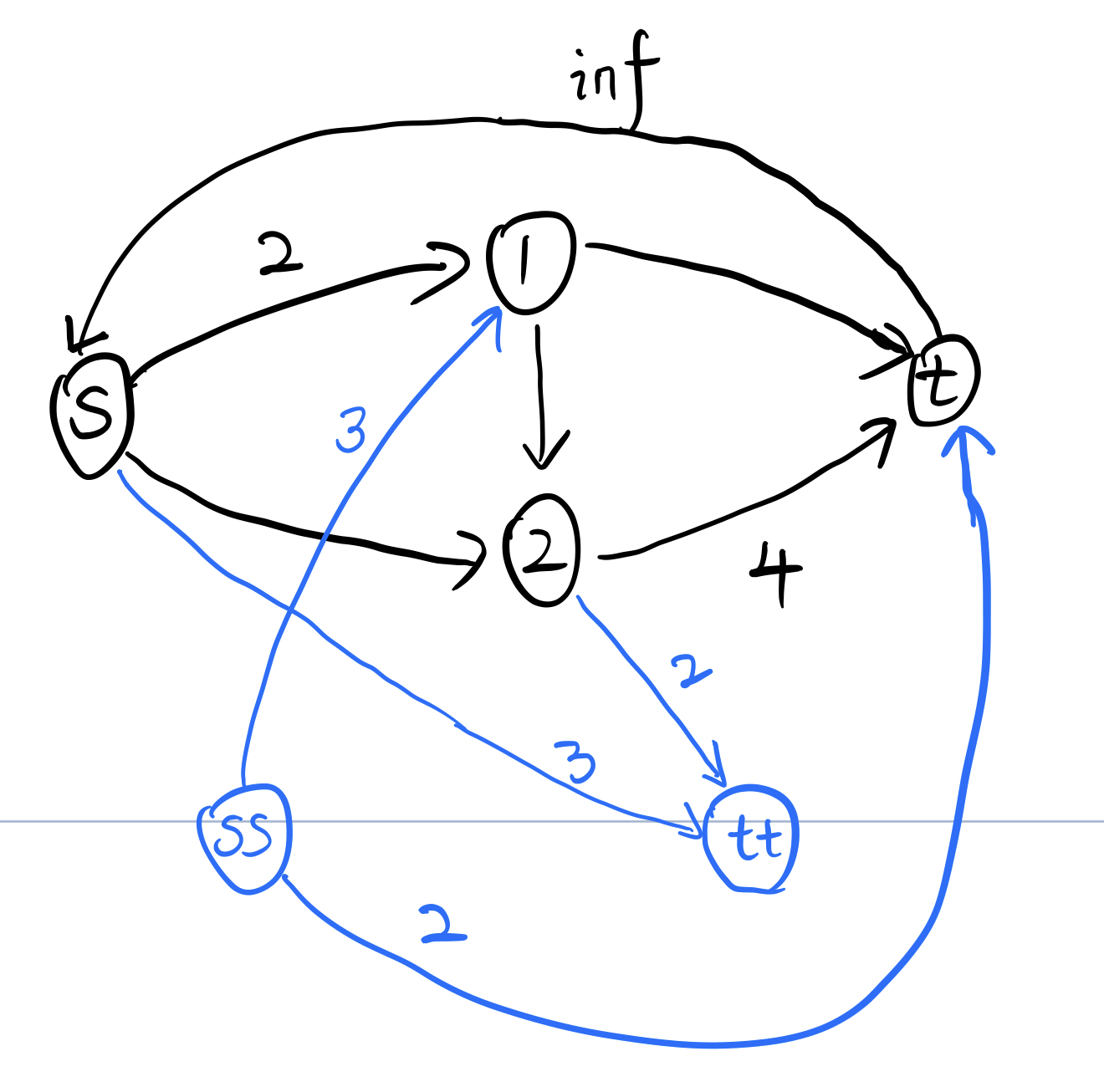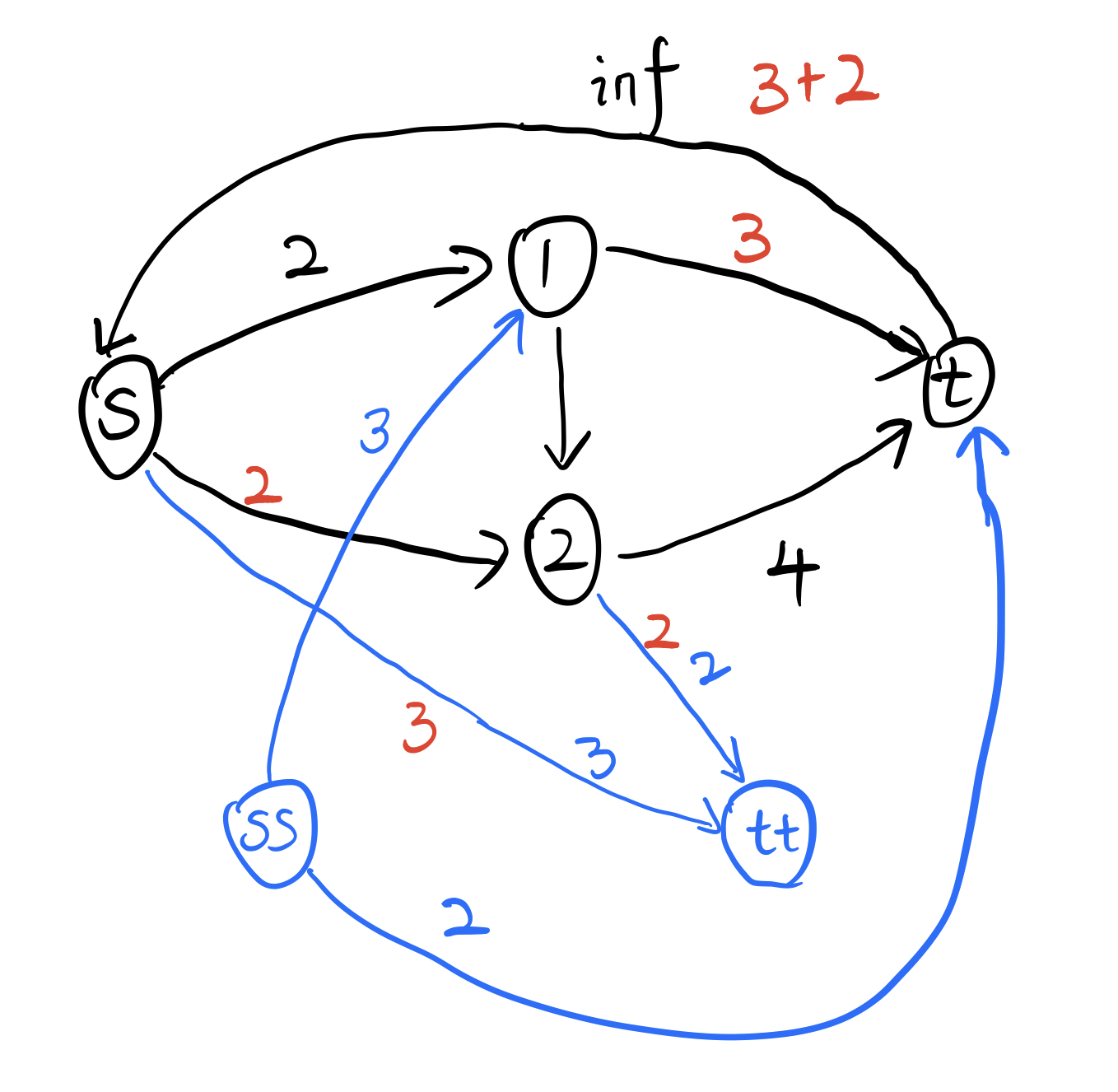$1$ 号点本可以直接走到 $2$ 点然后到达 $tt$ 点，但是它却经过了 $(t,s)$ 这条边绕了一圈才到 $tt$ 点。然而 $(t,s)$ 这条边的流量表示的是最后可行流的大小，所以想要获得最小流，那么应该让通过 $(t,s)$ 边的流量尽可能地少。

### 最大权闭合子图

• $s$ 向所有正权点连边，容量为权值。
• 所有负权点向 $t$ 连边，容量为权值的绝对值。
• 其他的边正常连，容量为正无穷。
• 跑 $s$ 到 $t$ 的最大流，答案为所有正权点的权值和减去最大流。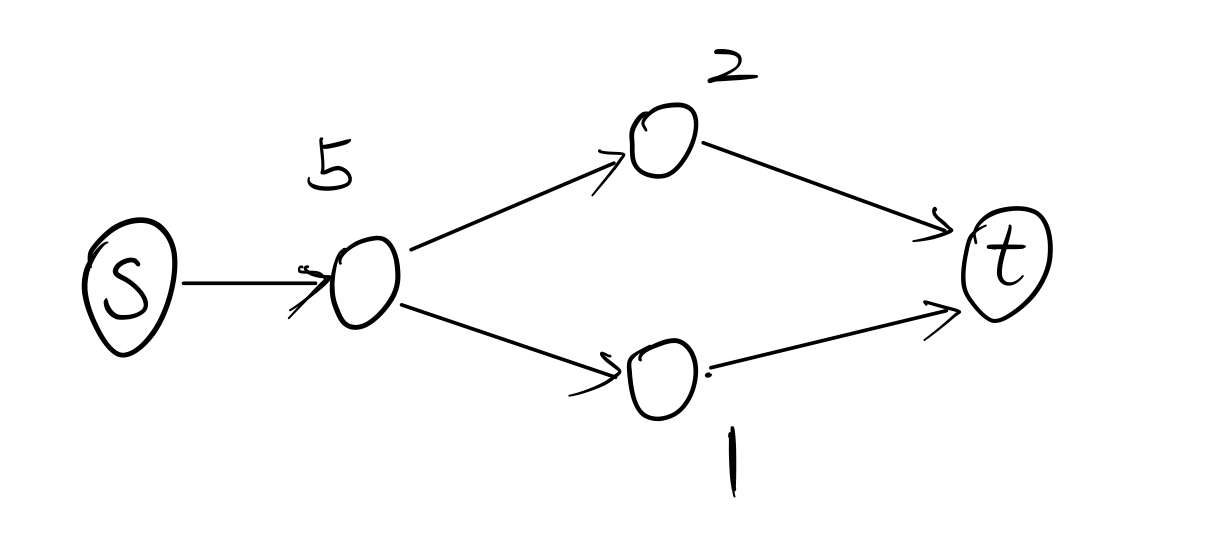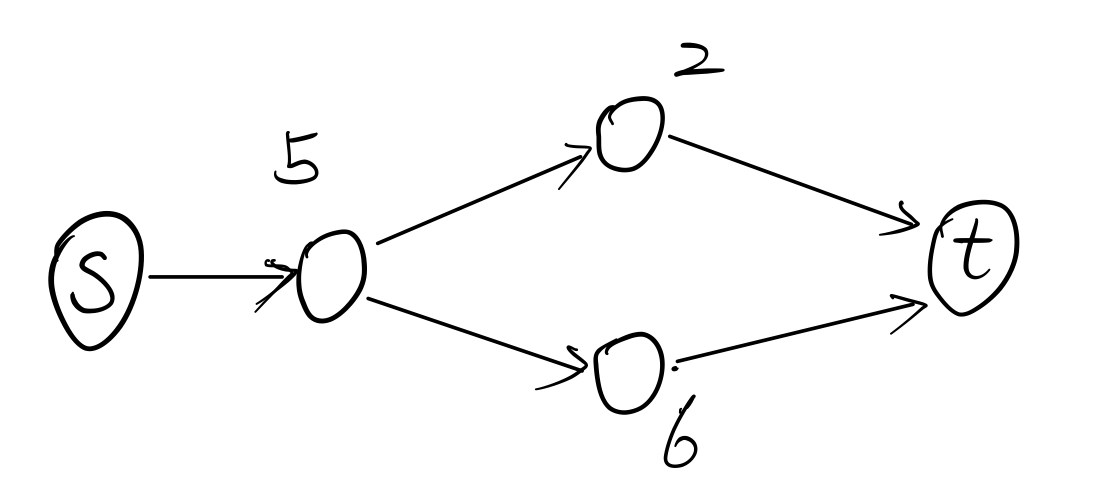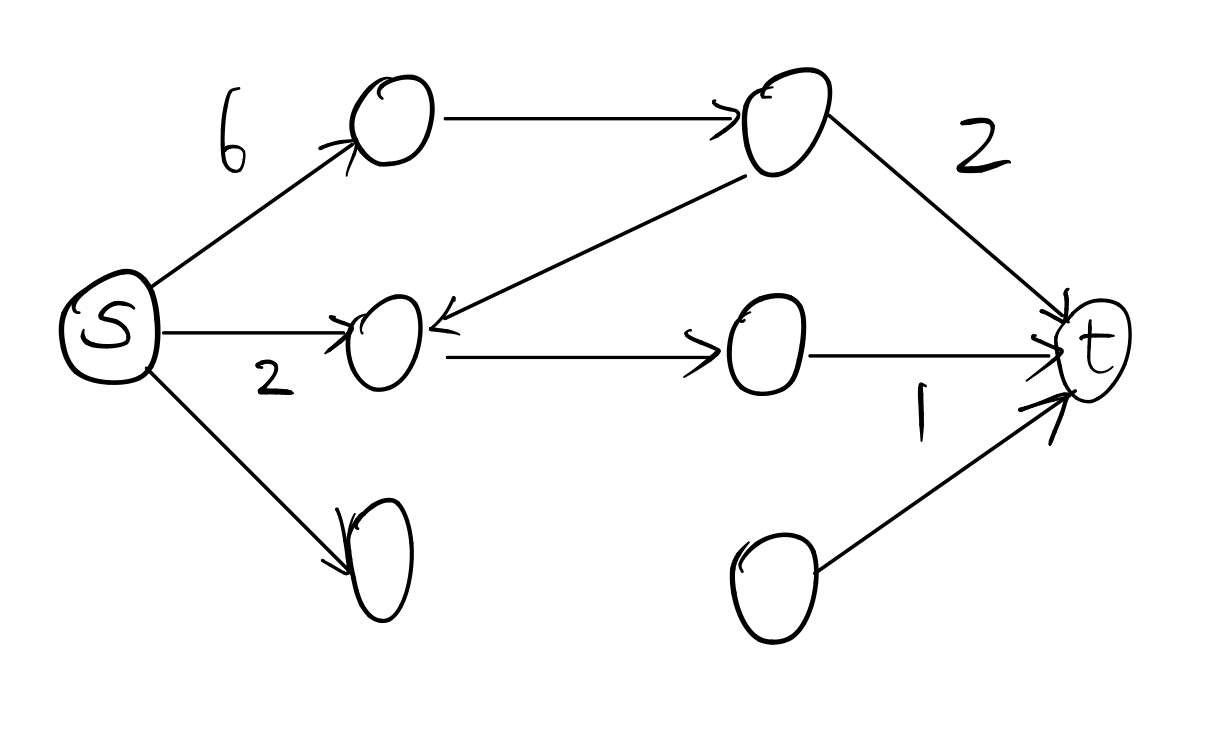### 最大密度子图

$$D = \frac{|E|}{|V|}$$

h(g) < 0  ->  g > D
h(g) > 0  ->  g < D

• 源点向每条边建立容量为 $1$ 的边。
• 每条边向其两个顶点建立无穷大的边。
• 每个顶点向汇点建立容量为 $g$ 的边。
• 跑 $s$ 到 $t$ 的最大流，答案为 $|E| - flow$。

### 拆点与拆边

#### 拆边

##### 例题

$n$ 个城市，$m$ 条有向路，现在要运送 $k$ 个货物从 $1$ 号城市到 $n$ 号城市。每条路有个系数 $a_i$，和一个流量上限 $c_i$，运送 $x$ 个货物的花费为 $a_ix^2$。现在求运送完成的最小花费。

$c_i \leq 5$